GRE Subject Test: Math : Defining Derivatives with Limits

Example Questions

Example Question #1 : Defining Derivatives With Limits

Evaluate: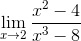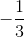Does Not ExistExplanation:

Step 1: Try plugging ininto the denominator of the function. We want to make sure that the bottom does not become...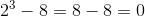.. We got zero, and we cannot have zero in the denominator. So, we must try and factor the function (numerator and denominator):

Step 2: Factor: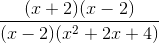Step 3: Reduce: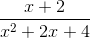Step 4: Now that we got rid of the factor that made the denominator zero, we know that this function has a limit.

Step 5: Plug ininto the reduced factor form:

Simplify as much as possible...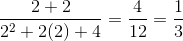The limit of this function as x approachesisAll GRE Subject Test: Math Resources